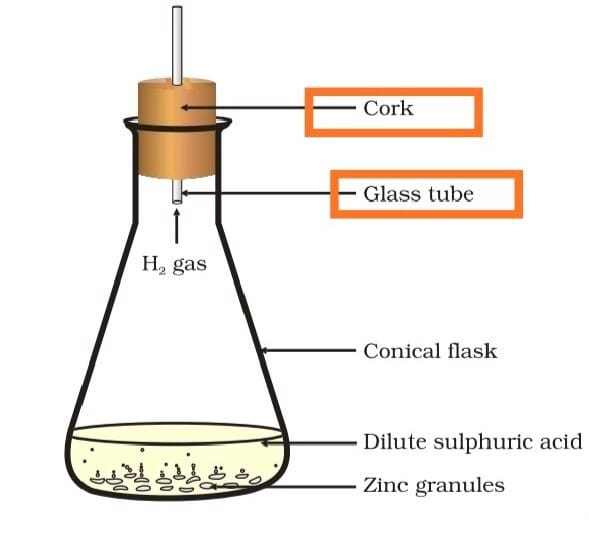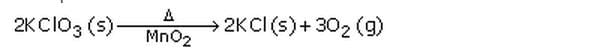Lakhmir Singh & Manjit Kaur Solutions: Chemical Reactions & Equations - 1

# Lakhmir Singh & Manjit Kaur Solutions: Chemical Reactions & Equations - 1 - Science Class 10

(Page No - 18)

Question 1:
Why is respiration considered an exothermic process?
Solution :

Respiration is an exothermic process because energy is produced during this process.

Question 2:
On what basis is a chemical equation balanced?
Solution :

A balanced chemical equation has an equal number of atoms of different elements in the reactants and
products.

Question 3:
What happens chemically when quicklime is added to water filled in a bucket?
Solution :

When quicklime is added to water, it forms slaked lime along with the evolution of heat. There will be a rise in the temperature of the bucket.

Question 4:
Why should magnesium ribbon be cleaned before burning in air?
Solution :

Magnesium ribbon
should be cleaned before burning in the air to remove the protective layer of
basic magnesium carbonate from its surface.

Question 5:
State whether the following statement is true or false :
A chemical equation can be balanced easily by altering the formula of a reactant or product.
Solution :

False.

Question 6:
What is wrong with the following chemical equation?
Mg + O ——— > MgO
Correct and balance it.
Solution :

Oxygen should be in molecular form, O2
2Mg + O2 —–> 2MgO

Question 7:
What does the symbol (aq) represent in a chemical equation?
Solution :

The symbol (aq)
represents an aqueous solution in a chemical equation.

Question 8:
Why is photosynthesis considered an endothermic reaction?
Solution :

Photosynthesis is an
endothermic reaction because sunlight energy is absorbed by green plants
during this process.

Question 9:
How will you indicate the following effects in a chemical equation?
(a) A solution made in water
(b) Exothermic reaction
(c) Endothermic reaction
Solution :

(a) Aqueous solution is indicated by the symbol ‘aq’.
An exothermic reaction is indicated by writing “+Heat” or “+Heat energy”  or “+Energy” on the product's side of an equation.
An endothermic reaction is indicated by writing “+Heat” or “+Heat energy”  or “+Energy” on the reactants side of an equation.

Question 10:
Translate the following statements into chemical equations and then balance the equations :
(a) Hydrogen sulphide gas burns in the air to give water and sulphur dioxide.
(b) Phosphorus burns in oxygen to give phosphorus pentoxide.
(c) Carbon disulphide burns in the air to give carbon dioxide and sulphur dioxide.
(d) Aluminium metal replaces iron from ferric oxide, Fe2O3, giving aluminium oxide and iron.
(e) Barium chloride reacts with zinc sulphate to give zinc chloride and barium sulphate.
Solution :

(a) 2H2S + 3O2 —–> 2H2O + 2SO2
(b) P+ 5O2 —–> 2P2O5
(c) CS2 + 3O2 —–> CO+ 2SO2
(d) 2Al + Fe2O3 —–> Al2O3 + 2Fe
(e) BaCl+ ZnSO4 —–> ZnCl+ BaSO4

Question 11:
Write the balanced chemical equations for the following reactions :
(a) Calcium hydroxide + Carbon dioxide ——– > Calcium carbonate + Water
(b) Aluminium + Copper chloride ——– > Aluminium chloride + Copper
Solution :

(a) Ca(OH)+ CO2 —–> CaCO3 + H2O
2Al + 3CuCl2 —–> 2AlCl3 + 3Cu

(Page No - 19)

Question 12:
Complete and balance the following equations :
(a) NaOH + ………… —–> Na2S04 + H20
(b) Ca(OH)2 + ……….—–> CaC03 + H20
Solution :

(a) 2NaOH + H2SO4 —–> Na2SO4 + 2H2O
(b) Ca(OH)+ CO2 —–> CaCO3 + H2O

Question 13:
Correct and balance the following equations :

1. Ca + H20 — > CaOH + H
2. N + H — > NH3

Solution :

1. Ca + 2H2O —–> Ca(OH)2 + H2
2. N2 + 3H—–> 2NH3

Question 14:
Write complete balanced equations for the following reactions :
(a) Calcium (solid) + Water (liquid) —–> Calcium hydroxide (solution) + Hydrogen (gas)
(b) Sulphur dioxide (gas) + Oxygen (gas) —–> Sulphur trioxide (gas)
Solution :

(a) Ca (s) + 2H2O(l) —–> Ca(OH)2(aq) + H(g)
(b) 2SO2(g) + O2 (g) —–> 2SO3(g)

Question 15:

1. Na + O2 —–> Na2O
2. H2O—–> H2O + O2
3. Mg(OH)2+ HCl —–> MgCl2 + H2O.
4. Fe + O—–> Fe2O3
5. Al(OH)3 —–> Al2O3 + H2O
6. NH3+ CuO —–> Cu + N+ H2O
7. Al2(SO4)3+ NaOH —–> Al(OH)3 + Na2SO4
8. HNO3+ Ca(OH)2 —–> Ca(NO3)2 + H2O
9. NaOH + H2SO4 —–> Na2SO4 + H2O
10. BaCl2+ H2SO4 —–> BaSO4 + HCl

Solution :

1. 4Na + O2 —–> 2Na2O
2. 2H2O2 —–> 2H2O + O2
3. Mg(OH)2+ 2HCl —–> MgCl2 + 2H2O.
4. 4Fe + 3O—–> 2Fe2O3
5. 2Al(OH)3 —–> Al2O+ 3H2O
6. 2NH3+ 3CuO —–> 3Cu + N2 + 3H2O
7. Al2(SO4)3+ 6NaOH —–> 2Al(OH)3 + 3Na2SO4
8. 2HNO3+ Ca(OH)—–> Ca(NO3)2 + 2H2O
9. 2NaOH + H2SO4 —–> Na2SO4 + 2H2O
10. BaCl2+ H2SO4 —–> BaSO4 + 2HCl

Question 16:
Fill in the following blanks with suitable words :
(a) Chemical equations are balanced to satisfy the law of…….
(b) A solution made in water is known as an………. solution and indicated by the symbol…………….
Solution :

(a) Conservation of mass
(b) Aqueous; (aq)

Question 17:
(a) Give one example of a chemical reaction.
(b) State two characteristics of the chemical reaction which takes place when dilute sulphuric acid is poured over zinc granules.
(c) Give two characteristics of the chemical reaction which occurs on adding potassium iodide solution to lead nitrate solution.
Solution :

(a) Magnesium Ribbon is heated in the presence of air to form a white powder called magnesium oxide.
(b) When dilute sulphuric acid is poured over zinc granules

1. there will be a rise in the temperature
2. evolution of hydrogen gas.Formation of hydrogen gas

(c) A yellow precipitate is formed.

1. There will be a change in color (from colourless to yellow).

Question 18:
(a) What is a chemical equation? Explain with the help of an example.
(b) Giving examples, state the difference between balanced and unbalanced chemical equations.
(c) Balance the following chemical equations :

1. NH —–> N2 + H2
2. C +C02 —–> CO

Solution :
(a) The method of representing a chemical reaction with the help of symbols and formulae of substances involved in it is called a chemical equation.
Example: Zinc metal reacts with dilute sulphuric acid to form zinc sulphate and hydrogen gas. This equation is written as: Zn + H2SO—–> ZnSO4 + H2
(b) A balanced chemical equation has an equal number of atoms of different elements in the reactants and products. It has equal masses of various elements in the reactants and products.
Example: Zn + H2SO—–> ZnSO4 + H2
An unbalanced chemical equation has an unequal number of atoms of one or more elements in the reactants and products. It has unequal masses of various elements in the reactants and products.
Example: H2 + O2 —–> 2H2O
(c) 2NH3 —–> N2 + 3H2

1. C + CO2 —–> 2CO

Question 19:
When hydrogen is passed over copper oxide, copper and steam are formed. Write a balanced equation for this reaction and state which of the chemicals are :

1. elements
2. compounds
3. reactants
4. products
5. metals
6. non-metals

Solution :
H2 + CuO —–> Cu + H2O

1. Elements: H2 and Cu
2. Compounds: CuO and H2O
3. Reactants: H2 and CuO
4. Products: Cu
5. Metal: Cu
6. Non-metal: H2

Question 20:
(b) Write a balanced chemical equation from the following information :
An aqueous calcium hydroxide solution (lime water) reacts with carbon dioxide gas to produce a solid calcium carbonate precipitate and water.
Solution :

(i) By indicating the physical states of the reactants and products. Example: Gaseous state is indicated by the symbol (g).
Zn (s) + H2SO4 (aq) —–> ZnSO4 (aq) + H(g)
(ii) By indicating the heat changes taking place in the reaction. For xxample: An exothermic reaction is indicated by writing “+Heat” or “+Heat energy” or “+Energy” on the products side of an equation.
C (s) + O(g) —–> CO2 (g) + Heat
(iii) By indicating the “conditions” under which the reaction takes place.
Example: Delta stands for heat which is written over the arrow of the equation.(b) Ca(OH)(aq) + CO2 (g) —–> CaCO3 (s) + H2O (l)

Question 21:
(a) What is a balanced chemical equation? Why should chemical equations be balanced?
(b) Aluminium burns in chlorine to form aluminium chloride (AlCl3). Write a balanced chemical equation for this reaction.
(c) Potassium metal reacts with water to give potassium hydroxide and hydrogen gas. Write a balanced chemical equation for this reaction.
Solution :

(a) A balanced chemical equation has an equal number of atoms of different elements in the reactants and products. It has equal masses of various elements in the reactants and products. A chemical equation should be balanced to satisfy the law of conservation of chemical reactions.
(b) 2Al + 3Cl2 —–> 2AlCl3
(c) 2K + 2H2O —–> 2KOH + H2

The document Lakhmir Singh & Manjit Kaur Solutions: Chemical Reactions & Equations - 1 | Science Class 10 is a part of the Class 10 Course Science Class 10.
All you need of Class 10 at this link: Class 10

## Science Class 10

78 videos|509 docs|153 tests

## FAQs on Lakhmir Singh & Manjit Kaur Solutions: Chemical Reactions & Equations - 1 - Science Class 10

 1. What are chemical reactions?Ans. Chemical reactions are processes in which substances undergo a transformation to form new substances with different properties. It involves the breaking and formation of chemical bonds between atoms, resulting in the rearrangement of atoms to create new molecules.
 2. How are chemical equations written?Ans. Chemical equations are written using chemical symbols and formulas to represent the reactants and products involved in a chemical reaction. The reactants are written on the left side of the equation, separated by a plus sign (+), while the products are written on the right side, separated by an arrow (→).
 3. What is the importance of balancing chemical equations?Ans. Balancing chemical equations is important because it ensures that the law of conservation of mass is obeyed. This law states that matter cannot be created or destroyed in a chemical reaction, only rearranged. By balancing the equation, we ensure that the number of atoms of each element is the same on both sides of the equation.
 4. How can we identify chemical reactions?Ans. There are several ways to identify chemical reactions. Some common signs include the formation of a precipitate (a solid that forms from a solution), the evolution of a gas (bubbles or a change in odor), a color change, or the release or absorption of energy (heat or light).
 5. What are the different types of chemical reactions?Ans. There are several types of chemical reactions, including combination reactions (two or more substances combine to form a new substance), decomposition reactions (a single substance breaks down into two or more simpler substances), displacement reactions (one element replaces another in a compound), and redox reactions (involving the transfer of electrons between species). These types can further be classified into various subcategories.

## Science Class 10

78 videos|509 docs|153 testsExplore Courses for Class 10 examSignup to see your scores go up within 7 days! Learn & Practice with 1000+ FREE Notes, Videos & Tests.
10M+ students study on EduRev
Track your progress, build streaks, highlight & save important lessons and more!
Related Searches

,

,

,

,

,

,

,

,

,

,

,

,

,

,

,

,

,

,

,

,

,

;